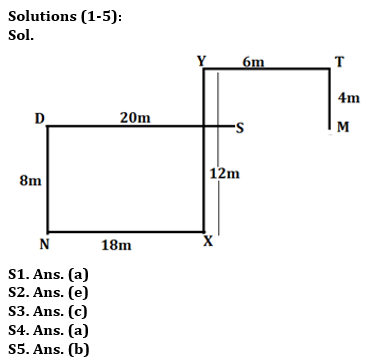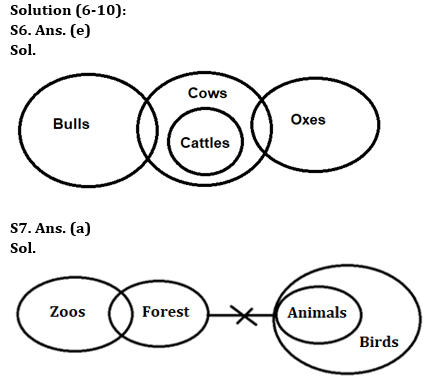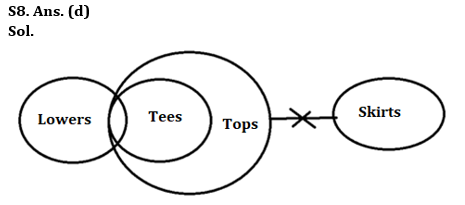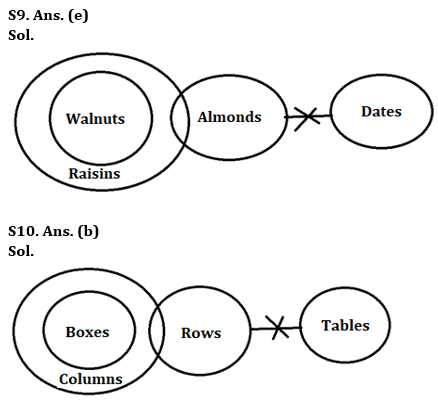Latest Banking jobs   »

# Reasoning Ability Quiz For Bank Foundation 2023 -02nd June

Directions (1-5): Study the information carefully and answer the questions given below.
Amit starts his journey from point S, walks 20m towards west to reach at point D, then takes a left turn and walks for 8m to reach point N. From point N he takes a left turn and walks for 18m to reach at point X. From point X, he takes a left turn and walks 12m to reach at point Y, then takes a right turn and walks 6m to reach point T. From point T, He walks 4m in south direction and reached point M.

Q1. What is the shortest distance between point S and M?
(a) 4m
(b) 6m
(c) 2m
(d) 5m
(e) None of these

Q2. If point H is 6m west of point M then in which direction is point Y with respect to point H?
(a) South
(b) North-west
(c) South-east
(d) East
(e) North

Q3. In which direction is point T with respect of D?
(a) North
(b) South-west
(c) North-east
(d) East
(e) South-east

Q4. What is the shortest distance between Point D and Point X?
(a) √388m
(b) 10m
(c) 15m
(d) 20m
(e) None of these

Q5. In which direction is point N with respect of point Y?
(a) North-east
(b) South-west
(c) North-west
(d) South
(e) None of these

Directions (6-10): In the question below, three statements are given followed by the conclusions. You have to take the given statements to be true even if they seem to be at variance with commonly known facts. Read all the conclusions and then decide which of the given conclusions logically follows from the given statements disregarding commonly known facts.

Q6. Statements:
Only a few Bulls are Cows.
Some Oxes are Cows.
Only Cows are Cattles.
Conclusions:
I. Some Bulls are Cattles.
II. All Cows can be Bulls.
(a) If only conclusion I follows
(b) If only conclusion II follows
(c) If either conclusion I or conclusion II follows
(d) If both conclusions I and II follow
(e) If neither conclusion I nor conclusion II follows

Q7. Statements:
Only a few Zoos are Forests.
No Forests are Animals.
All Animals are Birds.
Conclusions:
I. Some Zoos are not Forests.
II. Some Animals are not Zoos.
(a) If only conclusion I follows
(b) If only conclusion II follows
(c) If either conclusion I or conclusion II follows
(d) If both conclusions I and II follow
(e) If neither conclusion I nor conclusion II follows

Q8. Statements:
Some Lowers are Tees.
All Tees are Tops.
No Tops are Skirts.
Conclusions:
I. Some Lowers are not Skirts
II. No Skirts are Tees
(a) If only conclusion I follows
(b) If only conclusion II follows
(c) If either conclusion I or conclusion II follows
(d) If both conclusions I and II follow
(e) If neither conclusion I nor conclusion II follows

Q9. Statements:
All Walnuts are Raisins.
Some Raisins are Almonds.
No Almonds are Dates.
Conclusions:
I. Some Raisins are not Almonds.
II. Some Walnuts are Dates.
(a) If only conclusion I follows
(b) If only conclusion II follows
(c) If either conclusion I or conclusion II follows
(d) If both conclusions I and II follow
(e) If neither conclusion I nor conclusion II follows

Q10. Statements:
All Boxes are Columns.
Some Columns are Rows.
No Rows are Tables.
Conclusions:
I. No Boxes are Tables
II. Some Columns are not Tables
(a) If only conclusion I follows
(b) If only conclusion II follows
(c) If either conclusion I or conclusion II follows
(d) If both conclusions I and II follow
(e) If neither conclusion I nor conclusion II follows

Solutions## FAQs

### What is the selection process of the Bank Clerk?

The selection process of the Bank Clerk is Prelims & Mains.

#### Congratulations!Union Budget 2023-24: Free PDF